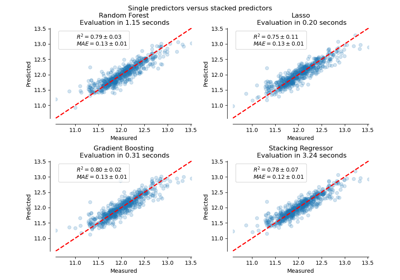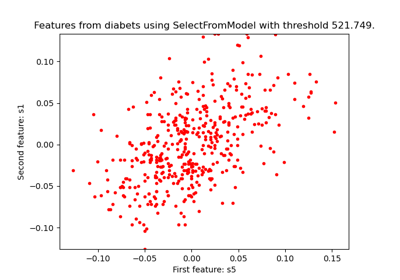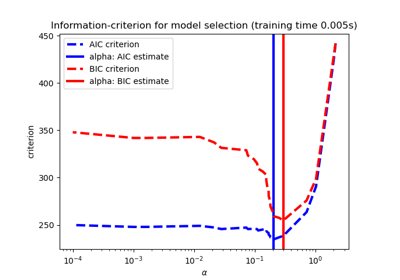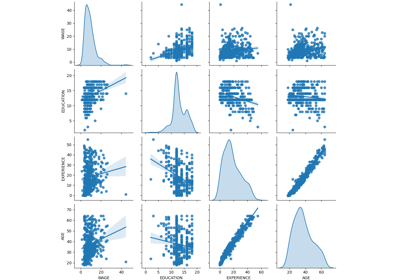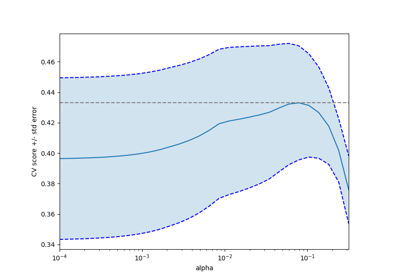# 3.2.4.1.3. sklearn.linear_model.LassoCV¶

class sklearn.linear_model.LassoCV(*, eps=0.001, n_alphas=100, alphas=None, fit_intercept=True, normalize=False, precompute='auto', max_iter=1000, tol=0.0001, copy_X=True, cv=None, verbose=False, n_jobs=None, positive=False, random_state=None, selection='cyclic')[source]

Lasso linear model with iterative fitting along a regularization path.

See glossary entry for cross-validation estimator.

The best model is selected by cross-validation.

The optimization objective for Lasso is:

(1 / (2 * n_samples)) * ||y - Xw||^2_2 + alpha * ||w||_1


Read more in the User Guide.

Parameters
epsfloat, default=1e-3

Length of the path. eps=1e-3 means that alpha_min / alpha_max = 1e-3.

n_alphasint, default=100

Number of alphas along the regularization path

alphasndarray, default=None

List of alphas where to compute the models. If None alphas are set automatically

fit_interceptbool, default=True

whether to calculate the intercept for this model. If set to false, no intercept will be used in calculations (i.e. data is expected to be centered).

normalizebool, default=False

This parameter is ignored when fit_intercept is set to False. If True, the regressors X will be normalized before regression by subtracting the mean and dividing by the l2-norm. If you wish to standardize, please use sklearn.preprocessing.StandardScaler before calling fit on an estimator with normalize=False.

precompute‘auto’, bool or array-like of shape (n_features, n_features), default=’auto’

Whether to use a precomputed Gram matrix to speed up calculations. If set to 'auto' let us decide. The Gram matrix can also be passed as argument.

max_iterint, default=1000

The maximum number of iterations

tolfloat, default=1e-4

The tolerance for the optimization: if the updates are smaller than tol, the optimization code checks the dual gap for optimality and continues until it is smaller than tol.

copy_Xbool, default=True

If True, X will be copied; else, it may be overwritten.

cvint, cross-validation generator or iterable, default=None

Determines the cross-validation splitting strategy. Possible inputs for cv are:

• None, to use the default 5-fold cross-validation,

• int, to specify the number of folds.

• An iterable yielding (train, test) splits as arrays of indices.

For int/None inputs, KFold is used.

Refer User Guide for the various cross-validation strategies that can be used here.

Changed in version 0.22: cv default value if None changed from 3-fold to 5-fold.

verbosebool or int, default=False

Amount of verbosity.

n_jobsint, default=None

Number of CPUs to use during the cross validation. None means 1 unless in a joblib.parallel_backend context. -1 means using all processors. See Glossary for more details.

positivebool, default=False

If positive, restrict regression coefficients to be positive

random_stateint, RandomState instance, default=None

The seed of the pseudo random number generator that selects a random feature to update. Used when selection == ‘random’. Pass an int for reproducible output across multiple function calls. See Glossary.

selection{‘cyclic’, ‘random’}, default=’cyclic’

If set to ‘random’, a random coefficient is updated every iteration rather than looping over features sequentially by default. This (setting to ‘random’) often leads to significantly faster convergence especially when tol is higher than 1e-4.

Attributes
alpha_float

The amount of penalization chosen by cross validation

coef_ndarray of shape (n_features,) or (n_targets, n_features)

parameter vector (w in the cost function formula)

intercept_float or ndarray of shape (n_targets,)

independent term in decision function.

mse_path_ndarray of shape (n_alphas, n_folds)

mean square error for the test set on each fold, varying alpha

alphas_ndarray of shape (n_alphas,)

The grid of alphas used for fitting

dual_gap_float or ndarray of shape (n_targets,)

The dual gap at the end of the optimization for the optimal alpha (alpha_).

n_iter_int

number of iterations run by the coordinate descent solver to reach the specified tolerance for the optimal alpha.

Notes

For an example, see examples/linear_model/plot_lasso_model_selection.py.

To avoid unnecessary memory duplication the X argument of the fit method should be directly passed as a Fortran-contiguous numpy array.

Examples

>>> from sklearn.linear_model import LassoCV
>>> from sklearn.datasets import make_regression
>>> X, y = make_regression(noise=4, random_state=0)
>>> reg = LassoCV(cv=5, random_state=0).fit(X, y)
>>> reg.score(X, y)
0.9993...
>>> reg.predict(X[:1,])
array([-78.4951...])


Methods

 fit(X, y) Fit linear model with coordinate descent get_params([deep]) Get parameters for this estimator. path(X, y, *[, eps, n_alphas, alphas, …]) Compute Lasso path with coordinate descent Predict using the linear model. score(X, y[, sample_weight]) Return the coefficient of determination R^2 of the prediction. set_params(**params) Set the parameters of this estimator.
__init__(*, eps=0.001, n_alphas=100, alphas=None, fit_intercept=True, normalize=False, precompute='auto', max_iter=1000, tol=0.0001, copy_X=True, cv=None, verbose=False, n_jobs=None, positive=False, random_state=None, selection='cyclic')[source]

Initialize self. See help(type(self)) for accurate signature.

fit(X, y)[source]

Fit linear model with coordinate descent

Fit is on grid of alphas and best alpha estimated by cross-validation.

Parameters
X{array-like, sparse matrix} of shape (n_samples, n_features)

Training data. Pass directly as Fortran-contiguous data to avoid unnecessary memory duplication. If y is mono-output, X can be sparse.

yarray-like of shape (n_samples,) or (n_samples, n_targets)

Target values

get_params(deep=True)[source]

Get parameters for this estimator.

Parameters
deepbool, default=True

If True, will return the parameters for this estimator and contained subobjects that are estimators.

Returns
paramsmapping of string to any

Parameter names mapped to their values.

static path(X, y, *, eps=0.001, n_alphas=100, alphas=None, precompute='auto', Xy=None, copy_X=True, coef_init=None, verbose=False, return_n_iter=False, positive=False, **params)[source]

Compute Lasso path with coordinate descent

The Lasso optimization function varies for mono and multi-outputs.

(1 / (2 * n_samples)) * ||y - Xw||^2_2 + alpha * ||w||_1


(1 / (2 * n_samples)) * ||Y - XW||^2_Fro + alpha * ||W||_21


Where:

||W||_21 = \sum_i \sqrt{\sum_j w_{ij}^2}


i.e. the sum of norm of each row.

Read more in the User Guide.

Parameters
X{array-like, sparse matrix} of shape (n_samples, n_features)

Training data. Pass directly as Fortran-contiguous data to avoid unnecessary memory duplication. If y is mono-output then X can be sparse.

y{array-like, sparse matrix} of shape (n_samples,) or (n_samples, n_outputs)

Target values

epsfloat, default=1e-3

Length of the path. eps=1e-3 means that alpha_min / alpha_max = 1e-3

n_alphasint, default=100

Number of alphas along the regularization path

alphasndarray, default=None

List of alphas where to compute the models. If None alphas are set automatically

precompute‘auto’, bool or array-like of shape (n_features, n_features), default=’auto’

Whether to use a precomputed Gram matrix to speed up calculations. If set to 'auto' let us decide. The Gram matrix can also be passed as argument.

Xyarray-like of shape (n_features,) or (n_features, n_outputs), default=None

Xy = np.dot(X.T, y) that can be precomputed. It is useful only when the Gram matrix is precomputed.

copy_Xbool, default=True

If True, X will be copied; else, it may be overwritten.

coef_initndarray of shape (n_features, ), default=None

The initial values of the coefficients.

verbosebool or int, default=False

Amount of verbosity.

return_n_iterbool, default=False

whether to return the number of iterations or not.

positivebool, default=False

If set to True, forces coefficients to be positive. (Only allowed when y.ndim == 1).

**paramskwargs

keyword arguments passed to the coordinate descent solver.

Returns
alphasndarray of shape (n_alphas,)

The alphas along the path where models are computed.

coefsndarray of shape (n_features, n_alphas) or (n_outputs, n_features, n_alphas)

Coefficients along the path.

dual_gapsndarray of shape (n_alphas,)

The dual gaps at the end of the optimization for each alpha.

n_iterslist of int

The number of iterations taken by the coordinate descent optimizer to reach the specified tolerance for each alpha.

Notes

For an example, see examples/linear_model/plot_lasso_coordinate_descent_path.py.

To avoid unnecessary memory duplication the X argument of the fit method should be directly passed as a Fortran-contiguous numpy array.

Note that in certain cases, the Lars solver may be significantly faster to implement this functionality. In particular, linear interpolation can be used to retrieve model coefficients between the values output by lars_path

Examples

Comparing lasso_path and lars_path with interpolation:

>>> X = np.array([[1, 2, 3.1], [2.3, 5.4, 4.3]]).T
>>> y = np.array([1, 2, 3.1])
>>> # Use lasso_path to compute a coefficient path
>>> _, coef_path, _ = lasso_path(X, y, alphas=[5., 1., .5])
>>> print(coef_path)
[[0.         0.         0.46874778]
[0.2159048  0.4425765  0.23689075]]

>>> # Now use lars_path and 1D linear interpolation to compute the
>>> # same path
>>> from sklearn.linear_model import lars_path
>>> alphas, active, coef_path_lars = lars_path(X, y, method='lasso')
>>> from scipy import interpolate
>>> coef_path_continuous = interpolate.interp1d(alphas[::-1],
...                                             coef_path_lars[:, ::-1])
>>> print(coef_path_continuous([5., 1., .5]))
[[0.         0.         0.46915237]
[0.2159048  0.4425765  0.23668876]]

predict(X)[source]

Predict using the linear model.

Parameters
Xarray_like or sparse matrix, shape (n_samples, n_features)

Samples.

Returns
Carray, shape (n_samples,)

Returns predicted values.

score(X, y, sample_weight=None)[source]

Return the coefficient of determination R^2 of the prediction.

The coefficient R^2 is defined as (1 - u/v), where u is the residual sum of squares ((y_true - y_pred) ** 2).sum() and v is the total sum of squares ((y_true - y_true.mean()) ** 2).sum(). The best possible score is 1.0 and it can be negative (because the model can be arbitrarily worse). A constant model that always predicts the expected value of y, disregarding the input features, would get a R^2 score of 0.0.

Parameters
Xarray-like of shape (n_samples, n_features)

Test samples. For some estimators this may be a precomputed kernel matrix or a list of generic objects instead, shape = (n_samples, n_samples_fitted), where n_samples_fitted is the number of samples used in the fitting for the estimator.

yarray-like of shape (n_samples,) or (n_samples, n_outputs)

True values for X.

sample_weightarray-like of shape (n_samples,), default=None

Sample weights.

Returns
scorefloat

R^2 of self.predict(X) wrt. y.

Notes

The R2 score used when calling score on a regressor uses multioutput='uniform_average' from version 0.23 to keep consistent with default value of r2_score. This influences the score method of all the multioutput regressors (except for MultiOutputRegressor).

set_params(**params)[source]

Set the parameters of this estimator.

The method works on simple estimators as well as on nested objects (such as pipelines). The latter have parameters of the form <component>__<parameter> so that it’s possible to update each component of a nested object.

Parameters
**paramsdict

Estimator parameters.

Returns
selfobject

Estimator instance.

## 3.2.4.1.3.1. Examples using sklearn.linear_model.LassoCV¶